login  home  contents  what's new  discussion  bug reports help  links  subscribe  changes  refresh  edit

 Submitted by : (unknown) at: 2007-11-17T22:06:58-08:00 (15 years ago) Name : Axiom Version : default friCAS-20090114 Axiom-20050901 OpenAxiom-20091012 OpenAxiom-20110220 OpenAxiom-Release-141 Category : Axiom Aldor Interface Axiom Compiler Axiom Library Axiom Interpreter Axiom Documentation Axiom User Interface building Axiom from source lisp system MathAction Doyen CD Reduce Axiom on Windows Axiom on Linux Severity : critical serious normal minor wishlist Status : open closed rejected not reproducible fix proposed fixed somewhere duplicate need more info Optional subject :   Optional comment :

For some reason, the following forgets about one summand:

fricas
(1) -> integrate(exp(-x^2)+exp(x)/x,x)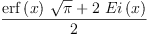(1)
Type: Union(Expression(Integer),...)

The problem is in expintegratepoly$INTTR. There you find the following definition:  -- returns either -- (q in GP, a in F) st p = q' + a, and a=0 or a has no integral in F -- or (q in GP, r in GP) st p = q' + r, and r has no integral elem/UP expintegratepoly(p, FRDE) == coef0:F := 0 notelm := answr := 0$GP
while p ^= 0 repeat
ans1 := FRDE(n := degree p, a := leadingCoefficient p)
answr := answr + monomial(ans1.ans, n)
if ~ans1.sol? then         -- Risch d.e. has no complete solution
missing := a - ans1.right
if zero? n then coef0 := missing
else notelm := notelm + monomial(missing, n)
p   := reductum p
zero? notelm => [answr, coef0]
[answr, notelm]


In principle, this function takes a polynomial p and tries to integrate every coefficient. If it finds an answer, it adds it to answr, otherwise to notelm. Note however, that the constant term of the p will never get added to notelm, even if it was not possible to integrate it. So maybe the last line should read:

      [answr, notelm+monomial(coef0, 0)]


This seems to "work", i.e., the integrals are then returned unevaluated. However, I'd rather have axiom to use the linearity of the integral... Note that

fricas
integrate(exp(-x^2)+sin(x),x)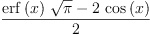(2)
Type: Union(Expression(Integer),...)

is an example for an integral where coef0 does not vanish but notelm does. So the more drastic change:

          if ~ans1.sol? then         -- Risch d.e. has no complete solution
missing := a - ans1.right
if zero? n then coef0 := missing
notelm := notelm + monomial(missing, n)
p   := reductum p
zero? notelm => [answr, coef0]
[answr, notelm]


is not necessary - but does not produce wrong results either.

By the way, here are some other - strange - manifestation of the same bug:

fricas
integrate(exp(-x^2)+1/x,x)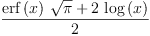(3)
Type: Union(Expression(Integer),...)
fricas
integrate(exp(x)/x+1/x,x)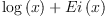(4)
Type: Union(Expression(Integer),...)

Although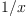is certainly elementary, and so is its integral, the bug manifests itself.

Martin

property change --kratt6, Sat, 20 Aug 2005 15:17:25 -0500 reply
Status: open => fix proposed

Name: #199 integratee^(x^2) => #199 #199 integrate(exp(-x^2)+exp(x)/x,x)

Name: #199 #199 integrate(exp(-x^2)+exp(x)/x,x) => #199 integrate(exp(-x^2)+exp(x)/x,x)

Status: fix proposed => closed

 Subject:   Be Bold !! ( 14 subscribers )